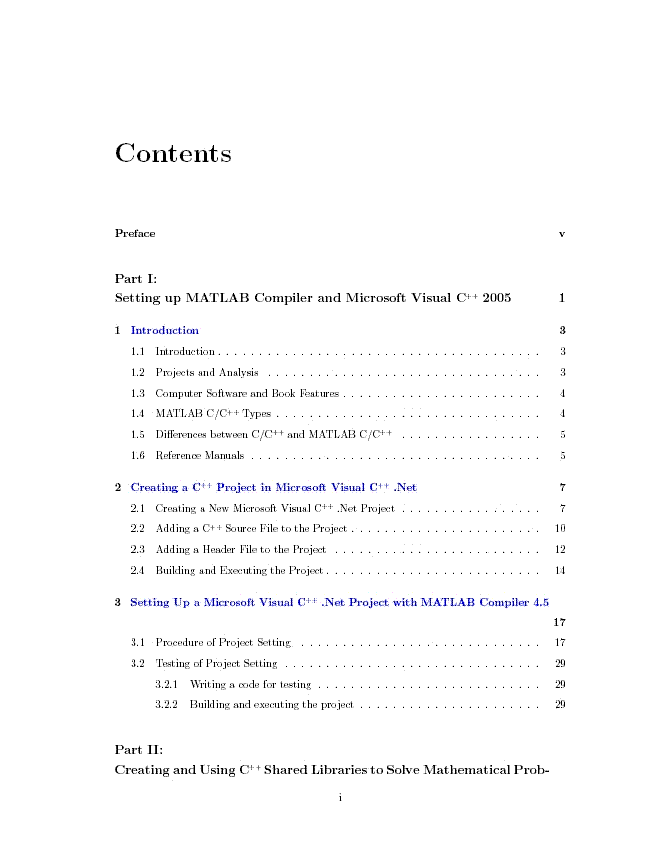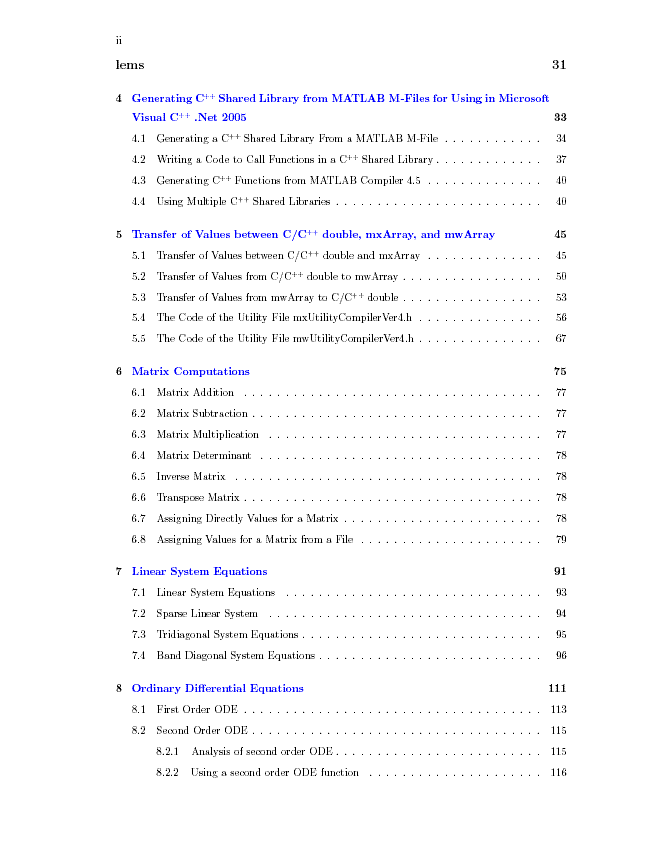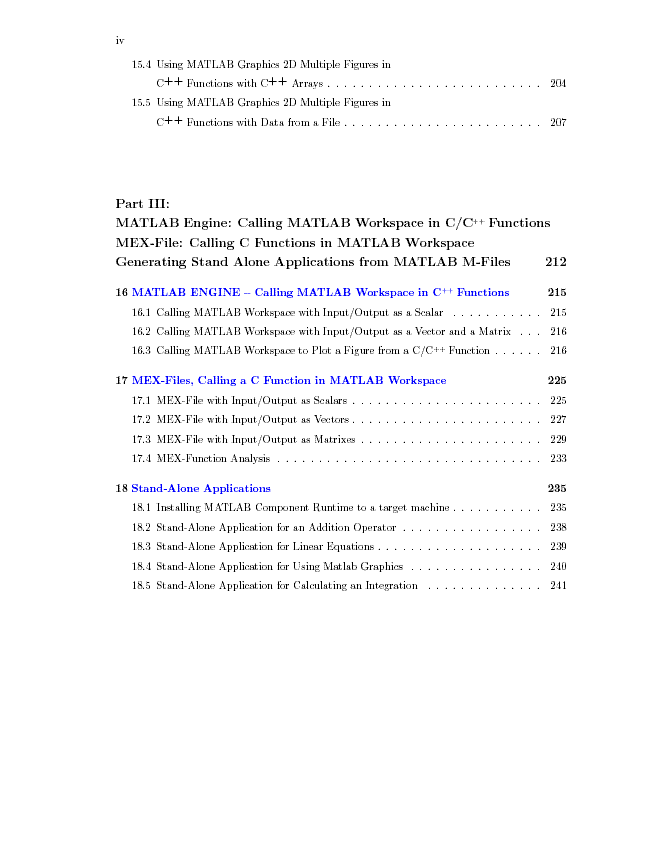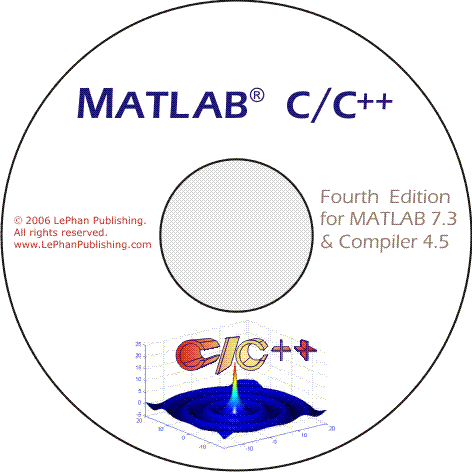MATLABÛ   C/C++  Book  for MATLAB Compiler 4.5

MATLAB C++ Book illustrates the works of C/C++ programming and MATLAB. The  book describes very detail how to use MATLAB built-in functions in C/C++ programming. If you are a C/C++ programmer and want to use the quality mathematical built-in functions in your C/C++ applications to solve mathematical problems in the C/C++ programming code, then this book is what you're looking for.

The features of this book are designed to handle four following projects:

1. Using MATLAB built-in math functions in C++ funtions.

2. Using the powerful MATLAB Graphics functions in C++ functions.

3. Calling MATLAB Workspace from C++ functions to  perform particular tasks then transfer back results to C++ functions.

4. Writing a MEX-function for existing C/C++ functions then call it from MATLAB Workspace.

5. Generating Stand Alone Application from existing M-files to use in a target machine which doesn't have MATLAB software.

In addition, the book has chapters to help you set up Microsoft Visual C++ .Net version 2005 with MATLAB Compiler 4.5. This MATLAB C/C++ Book is an ebook in the pdf format on CD-Rom which also includes complete C++ code of examples of 17 chapters . These example codes are developed, compiled, and tested in MATLAB 7.3 (R2006b) and MATLAB Compiler 4.5.

The C/C++ compiler used to test is Microsoft Visual C++ .Net version 2005. The examples are working on scalars, vectors, and matrixes which are inputs/outputs of functions for all applications. In addition, the example codes are portable and presented in the step-by-step method, therefore you can easily reuse the codes or write your own codes following the step-by-step procedure.

The MATLAB C/C++ Book is a great support for C/C++ programmers who are using MATLAB to develop applications and solutions. This book is reviewed by MATLAB staff and promoted at MATLAB Book Program and Technical Book Resources.

Using the combination of both tools, C/C++ and MATLAB, you have the best tool in your hand to solve the mathematical problems.

MATLAB and MATLAB Compiler are registered trademarks of The MathWork, Inc.
Microsoft, Windows, and Microsoft Visual C++ are